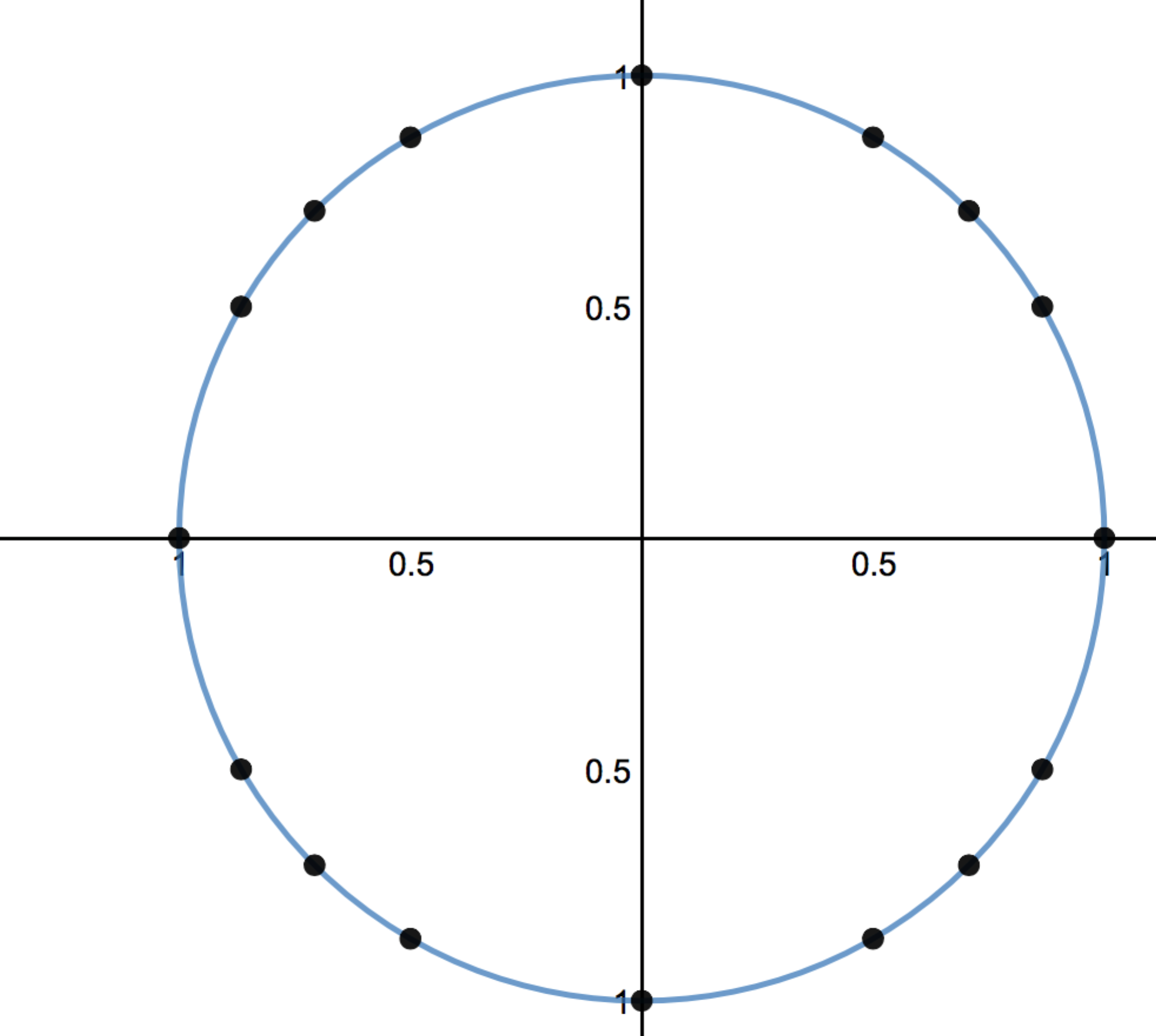# Right Triangles and Trigonometry

Lesson 15

Math

Unit 4

Lesson 15 of 19

## Objective

Use the first quadrant of the unit circle to define sine, cosine, and tangent values outside the first quadrant.

## Common Core Standards

### Core Standards

• F.TF.A.2 — Explain how the unit circle in the coordinate plane enables the extension of trigonometric functions to all real numbers, interpreted as radian measures of angles traversed counterclockwise around the unit circle.

• 8.G.A.1

## Criteria for Success

• Describe how the sine and cosine change depending on the quadrant you move to in the unit circle using reflections or rotations as a basis for the coordinate point changing.
• Describe the transformation necessary from $${\mathrm{sin}(x)}$$ to $$-{\mathrm{sin}(x)}$$ and from $${\mathrm{cos}(x)}$$ to $$-{\mathrm{cos}(x)}$$ as reflections over the $$x$$- and $$y$$-axes, respectively.
• Derive the unit circle by transforming the first quadrant reference angles across the axes.

## Tips for Teachers

• This lesson and the previous lesson cover key concepts in the unit by reinforcing and reviewing G-SRT.6 and G-SRT.7.
• The unit circle is used throughout calculus and is built on trigonometric ratios.
Fishtank Plus

Unlock features to optimize your prep time, plan engaging lessons, and monitor student progress.

## Anchor Problems

### Problem 1

Given a 30° angle on the unit circle,

a)  Find the coordinate point of this angle.
b)  Reflect this point over the y-axis.

### Problem 2

How is $${-\mathrm{sin}(45^\circ)}$$ different from $${\mathrm{sin}(-45^\circ)}$$? Use your unit circle to help determine this.

### Problem 3

Given a 60° angle on the unit circle,

1. Find the coordinate point of this angle.
2. Find $${\mathrm{sin}(60^\circ)}$$ and $${\mathrm{cos}(60^\circ)}$$.
3. How could you use the value of sine and cosine to find $${\mathrm{tan}(60^\circ)}$$?

## Problem Set

The following resources include problems and activities aligned to the objective of the lesson that can be used to create your own problem set.

• Include problems where students need to plot positive and negative angles on the unit circle. For example, “Which point on the unit circle marks -30°? What positive angle does this represent?”
• Include problems where students need to recreate the unit circle given only a few values.
• Include problems where students need to identify and correct an error with two trigonometric values that are congruent as well as two trigonometric values that are not congruent.
• Include problems where students are given either the point, the angle, or one value and need to name the other components.
• Include problems where students need to identify all of the angles that have a particular $$x$$- or $$y$$-coordinate on the unit circle. For example, “How many angles have an x-coordinate of $${\frac{1}{2}}$$? Name them and describe how you know that this is the case.”

Below is a unit circle.  The points marked on the circle represent the intersection of the terminal sides of the reference angles of 30°, 45°, and 60°. Write the coordinates for all of the marked points around the unit circle.#### References

Image

Unit Circle with Reference Triangles by Match Foundation, Inc. is made available by Desmos. Copyright © 2017 Desmos, Inc. Accessed March 13, 2017, 4:27 p.m..

Lesson 14

Lesson 16

## Lesson Map

Topic A: Right Triangle Properties and Side-Length Relationships

Topic B: Right Triangle Trigonometry

Topic C: Applications of Right Triangle Trigonometry

Topic D: The Unit Circle

Topic E: Trigonometric Ratios in Non-Right Triangles